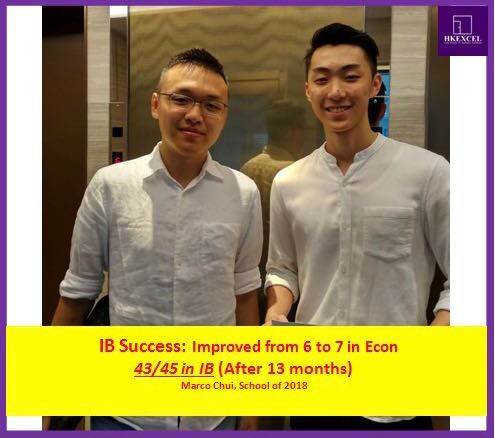Search

# IB Econ tutor, how can we help you get L7 in IB Econ?

IB Econ is one of the most popular subjects in the humanities. A lot of IB students strive for a Level 7, and hence many IB students are interested in getting a IB Econ tutor.

What are the key assessments in IB Econ that we can help you in?

The paper 1: There are 2 sections in the paper 1. One is for micro economics and one is for macro economics. Each section of 2 questions and students only need to pick one to answer. Each question will then consist of part A and B. Part A is a 10 mark question that normally involves explanation of certain economic concepts, whereas part B is a 15 mark question that involves evaluation. Students are required to answer these questions in essay format.

The paper 2: Similar to the paper 1, there are 2 sections in the paper 2- one for trade economics and one for developmental economics. Again, there are 2 questions in each section and students need to pick 1 of them to answer. The difference between paper 1 and paper 2 is that paper 2 is based on a newspaper article. Moreover, in a paper 2 question, there are typically 4 parts from part a to d. Part A involves defining economic terms, while part b and c involves explaining certain economic concepts, and part d involves evaluation of economic concepts.

The paper 3: the paper 3 is a paper that only HL students need to do. There are typically 3 questions and students need to pick 2 to tackle. Most questions in paper 3 involves calculations, but there are also some questions that requires you to explain certain economic concepts and writing.

The IA: Students are required to write 3 IAs, on different sections of the syllabus. The IA is essentially a 750word commentary on a newspaper article of the student’s choice. Typically, the student is required to explain economic theories in the article and then do an evaluation. The IA counts for 20% of the total score.

How can a tutor help?

Firstly, a good understanding of economic concepts is required to achieve top marks in the IB. Our tutors are focused on using lament terms to explain economic concepts, coupled with real world examples to help students easily grasp economic concepts in the IB syllabus.

Secondly, good question answering and essay writing skills are critical to scoring good grades in IB Econ. Our IB Econ tutor will teach students how to structure their answers and how to phrase their responses to get top grades.

Thirdly, one of the most difficult types of question for students to master are evaluation questions. Students often struggle with finding good arguments and presenting good arguments. Our IB Econ tutor teaches brainstorming frameworks that allows students to very quickly come up with good solid arguments for evaluation questions.

How does a class typically work?

In a typical class, our tutor will first make sure the student understands all concepts for a specific chapter. We do this by asking the student to explain concepts back to us, to see if they truly get it. We will then fill in knowledge gaps to ensure students fully understand the concepts.

Then, we will focus on teaching students how to answer questions for that particular chapter. We will give students an answering framework for each question and also give students some time to write sample essays. Our tutors will then read student’s essays and give constructive feedback to hep the students improve?

How do you help with the IA?

A lot of students seek help for the IA. For IA support, we use a tailor-made approach for each student, because each student has a different newspaper article to write a commentary on.One of the best IB econ miracles in 2018!

Our IB Econ tutor can help on all stages of the student’s IA depending on the student’s needs. If the student doesn't have an article chosen yet, our tutor can help the student to find good articles are the foundation of a good IA. If the student has an article already but is not sure how to write a commentary on, our tutors can help students to develop an essay outline. If the student already has an IA written, our student can also give constructive feedback to improve it for a Level 7.